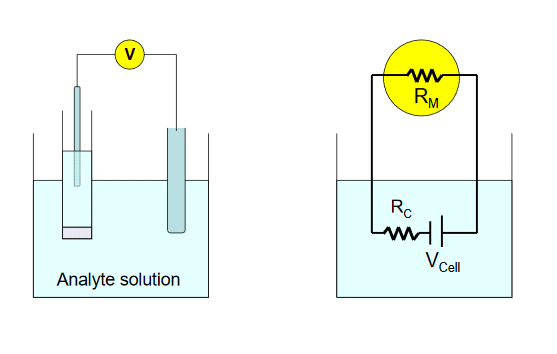# Instrumentation

$$\newcommand{\vecs}{\overset { \rightharpoonup} {\mathbf{#1}} }$$ $$\newcommand{\vecd}{\overset{-\!-\!\rightharpoonup}{\vphantom{a}\smash {#1}}}$$$$\newcommand{\id}{\mathrm{id}}$$ $$\newcommand{\Span}{\mathrm{span}}$$ $$\newcommand{\kernel}{\mathrm{null}\,}$$ $$\newcommand{\range}{\mathrm{range}\,}$$ $$\newcommand{\RealPart}{\mathrm{Re}}$$ $$\newcommand{\ImaginaryPart}{\mathrm{Im}}$$ $$\newcommand{\Argument}{\mathrm{Arg}}$$ $$\newcommand{\norm}{\| #1 \|}$$ $$\newcommand{\inner}{\langle #1, #2 \rangle}$$ $$\newcommand{\Span}{\mathrm{span}}$$ $$\newcommand{\id}{\mathrm{id}}$$ $$\newcommand{\Span}{\mathrm{span}}$$ $$\newcommand{\kernel}{\mathrm{null}\,}$$ $$\newcommand{\range}{\mathrm{range}\,}$$ $$\newcommand{\RealPart}{\mathrm{Re}}$$ $$\newcommand{\ImaginaryPart}{\mathrm{Im}}$$ $$\newcommand{\Argument}{\mathrm{Arg}}$$ $$\newcommand{\norm}{\| #1 \|}$$ $$\newcommand{\inner}{\langle #1, #2 \rangle}$$ $$\newcommand{\Span}{\mathrm{span}}$$$$\newcommand{\AA}{\unicode[.8,0]{x212B}}$$

You have realized by now that potentiometric measurements are fairly easy to make from the standpoint of instrumentation. In addition to the indicator electrode and the reference electrode, the only remaining component is a device used to measure the potential difference that exists between the two electrodes. If you have been with us to this point, you should remember that potentiometric measurements are ideally made under conditions of very little current flow. This means that the resistance (impedance to current flow) in the electrochemical cell must be very high (up to 100 MΩ). This is usually not a problem due to the nature of the indicator electrode, but the measurement of potential under these conditions requires the use of a device whose input resistance is even larger than the cell resistance.

When a voltmeter is introduced into the circuit, it brings with it a certain resistance, which changes the overall characteristics from those of the cell alone. That is, the very act of trying to make a measurement changes the quantity you set out to measure. In this context, the potential difference produced in the cell is not the quantity measured by the voltmeter. This perturbation is called the loading error, ER, and its value is given as % relative error by

$E_R = \dfrac{-R_C}{R_M + R_C} \times 100$

where RC is the resistance of the cell, and RM is the input resistance of the meter. In the diagram below, the circuit diagram on the right is a representation of the physical cell, shown on the left . The true cell potential arising from the analyte and its interaction with the sensing electrode is given by VCell.To achieve a loading error of - 0.1% for a cell resistance of 10 MΩ, an input impedance of 9990 MΩ (~10 GΩ) is required. Modern digital voltmeters utilizing operational amplifiers, which are high input resistance devices, are capable of easily achieving this level of accuracy in potential measurement.

Most of us recognize a “pH meter” when we see one. The measurement of pH is actually a measurement of potential at a glass membrane which is then converted to the pH scale. Most of these meters will also display potential in mV. These devices can be used with most types of the electrodes used in potentiometry, including other ion-selective electrodes, and electrodes utilizing enzyme membranes in their construction.

This page titled Instrumentation is shared under a CC BY-NC-SA 4.0 license and was authored, remixed, and/or curated by Contributor.